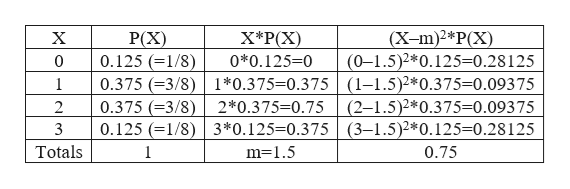# Hello I have to construct probability table using 3 coin toss and see how many head I can obtain.I used tree diagram and go the probability and obtain X (number of heads)P(X)01/813/823/831/8 Can you confirm that this is correct?X (number of heads)P(X)X*P(X)(X-m)2*P(X)00.12500.2812510.3750.3750.0937520.3750.750.0937530.1250.3750.28125Totals11.50.75

Question
3 views

Hello I have to construct probability table using 3 coin toss and see how many head I can obtain.

I used tree diagram and go the probability and obtain

 X (number of heads) P(X) 0 1/8 1 3/8 2 3/8 3 1/8

Can you confirm that this is correct?

 X (number of heads) P(X) X*P(X) (X-m)2*P(X) 0 0.125 0 0.28125 1 0.375 0.375 0.09375 2 0.375 0.75 0.09375 3 0.125 0.375 0.28125 Totals 1 1.5 0.75
check_circle

Step 1

It is given that, 3 coins are tossed.

The possible outcomes are {HHH, HHT, HTH, HTT, THH, THT, TTH, TTT}.

The formula for finding probability is shown below:

Step 2

In this case, the probability of getting 0 heads is 1/8.

The probability of getting 1 head is 3/8.

The probability of getting 2 heads is 3/8.

The probability of getting 3 heads is 1/8...help_outlineImage TranscriptioncloseX*P(X (X-m)2*P(X) (0-1.5)2*0.125=0.28 125 P(X) 0.125 (1/8) 0.375 (3/8) 1*0.375-0.375 (1-1.5)2*0.375-0.09375 0.375 (3/8) 0.125 1/8) 3*0.125-0.375 (3-1.5)2*0.125=0.28125 X 0*0.125-0 0 1 (2-1.5)2*0.375=0.093 75 2*0.375-0.75 2 Totals 0.75 m-1.5 fullscreen

### Want to see the full answer?

See Solution

#### Want to see this answer and more?

Solutions are written by subject experts who are available 24/7. Questions are typically answered within 1 hour.*

See Solution
*Response times may vary by subject and question.
Tagged in

### Statistics